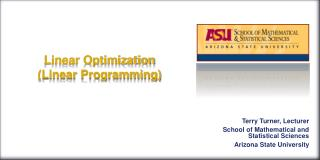DownloadDownload PresentationLinear Optimization (Linear Programming)

# Linear Optimization (Linear Programming)

Download Presentation## Linear Optimization (Linear Programming)

- - - - - - - - - - - - - - - - - - - - - - - - - - - E N D - - - - - - - - - - - - - - - - - - - - - - - - - - -
##### Presentation Transcript

1. Linear Optimization(Linear Programming) Terry Turner, Lecturer School of Mathematical and Statistical Sciences Arizona State University

2. Absolute Optimization • The domain is constrained to a closed and bounded region most of the time. Closed and bounded regions are guaranteed to have absolute extremes. • We analyze the partial derivatives for extremes within the region. • We analyze all paths for extremes. • We identify all corner points. • We evaluate everything we found. • The max is the highest z-value; • The min is the lowest z-value; • Discard anything in between.

3. The Basics is a linear function, called A criterion function, or An objective function. is optimized subject to constraints: All constraints are linear. Any inequality form regularly seen in math works. and usually with real world situations.

4. Example Maximize: Subject to:

5. Example Find the maximum and minimum values for Subject to: Assume and represent the supervisors required to manage factories X and Y respectively.. The objective function tells the output based on your time spent managing each factory. Since X is newer it takes half as many supervisors as Y. Assume and represent the times required to manage factories X and Y respectively.. The objective function tells the output based on your time spent managing each factory. Since X is newer it takes half as much of your time as Y.

6. Example A CEO invests some of his \$120,000 savings in an account paying 5% annual interest and the rest in another account paying 6% annual interest. • He invests at least \$60,000 in the account paying 6%. • The amount in the 6% account must be no more than three times the amount in the 5% account. How much must he invest in each account to maximize interest at the end of the year?

7. Linear Optimization Terry Turner, Lecturer School of Mathematical and Statistical Sciences Arizona State University